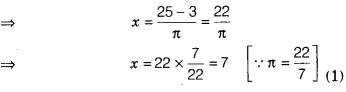# If πx + 3y = 25 and y = 1, then find x

If πx + 3y = 25 and y = 1, then find x.

Given, πx + 3y = 25
⇒πx + 3 x 1 = 25 [∵ y=1 given]